Q-Chem 4.3 User’s Manual

# 9.4 Minimum-Energy Crossing Points

Conical intersections are the regions of the potential energy surface characterized by degeneracy between two or more electronic states with the same symmetry. For a two-state intersection, the intersection consists of an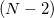-dimensional hypersurface (the seam space) within which the two states are degenerate, where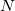is the number of internal coordinates of the molecule. (The remaining two dimensions for a branching space  in which the degeneracy is lifted by any infinitesimal displacement.) Radiationless transitions between the two electronic states are likely to occur in an around a conical seam. The first step in any study of nonadiabatic excited-state dynamics is often an exploration of the geometries and energies of the lowest-energy point within the seam space, which is the so-called minimum-energy crossing point  (MECP). In some sense, the MECP is to internal conversion and photochemical processes what the transition state is to single-surface chemical reactions.

The two-dimensional branching space between electronic states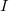and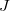is spanned by a pair of vectors that are usually denoted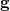and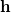: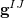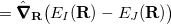(9.2)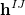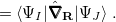(9.3)

While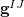is available analytically for any electronic structure method that has analytic excited-state gradients, analytic implementations of the nonadiabatic coupling vector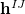are not routinely available. For this reason, several algorithms have been developed to optimize MECPs without the need to evaluate, and three such algorithms are available in Q-Chem. The first of these is a penalty-constrained optimization algorithm developed by Levine et al. , in which the objective function that is optimized to locate the MECP is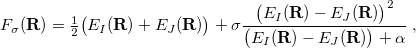(9.4)

whereis a fixed parameter to avoid singularities andis a Lagrange multiplier for a penalty function meant to drive the energy gap to zero. Optimization of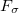proceeds iteratively for increasingly large values of the parameter. The second MECP optimization algorithm is a simplification of the first one that we call the “direct” method. Here, the gradient of the objective function is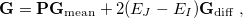(9.5)

where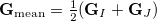(9.6)

is the mean energy gradient (with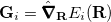being the nuclear gradient for state), and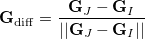(9.7)

is the normalized difference gradient. Finally,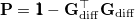(9.8)

projects the gradient difference direction out of the mean energy gradient in Eq. eq:direct_MECP. The third and final MECP optimization algorithm that is available in Q-Chem is the branching-plane updating method developed by Maeda et al. . This algorithm uses a gradient that is similar to that in Eq. eq:direct_MECP but projects out not just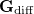in Eq. eq:MECP_projector but also a second vector that is orthogonal to it.

None of these three methods requires evaluation of nonadiabatic couplings, and all three can be used to optimize MECPs at the CIS, SF-CIS, TDDFT, SF-TDDFT, and SOS-CIS(D0) levels. The direct algorithm can also be used for EOM-XX-CCSD methods. Note, however, that all linear-response methods (a category that includes CIS, TDDFT, and EOM-XX-CCSD) incorrectly describe the topology of any conical intersection that involves the reference (usually, ground) state. Specifically, it can be shown in such cases that only a one-dimensional branching space is obtained . If conical intersections involving the ground state are to be described correctly, one must use a different reference state, which can be accomplished with spin-flip (SF) methods [420, 421, 422]. In particular, using a high-spin triplet reference state, the ground-state singlet (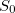) appears as an excitation (possibly with a negative excitation energy) alongside the excited-state singlets,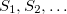, so there is no topology problem in describing an/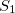conical intersection. Accurate MECP geometries, as compared to multireference configuration-interaction benchmarks, have been reported . It should be noted, however, that the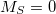component of the triplet state also shows up as an excitation in SF methods, and while Q-Chem attempts to identify this triplet state automatically (based on a threshold for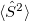), severe spin contamination can sometimes hamper one’s ability to distinguish this state from the singlet excited states .

In Q-Chem 4.3, analytic derivative couplings for CIS and TDDFT have been implemented 10.3. Thus, CIS and TDDFT MECP optimizations can use this new feature to reduce the optimization cycles. Note that the $derivative_coupling section is not required in MECP optimization jobs, and$rem variable MECP_METHODS must be set to BRANCHING_PLANE.

## 9.4.1 Job Control

MECP_OPT
 Determines whether we are doing MECP optimizations.

TYPE:
 LOGICAL

DEFAULT:
 FALSE

OPTIONS:
 TRUE Do MECP optimizations. FALSE Don’t do MECP optimizations.

RECOMMENDATION:
 None.

MECP_METHODS
 Determines which method to be used.

TYPE:
 STRING

DEFAULT:
 BRANCHING_PLANE

OPTIONS:
 BRANCHING_PLANE Use the branching-plane updating method. MECP_DIRECT Use the direct method. PENALTY_FUNCTION Use the penalty-constrained method.

RECOMMENDATION:
 The direct method is stable for small molecules or molecules with high symmetries, but the branching-plane updating method is more efficient for larger molecules. However, the latter does not work if the two states have different symmetries. If using branching plane updating method, GEOM_OPT_COORDS must be set to 0 in the $rem section (i.e., this algorithm is available in Cartesian coordinates only). The penalty-constrained method converges slowly and is suggested only when the other methods do not work. MECP_STATE1  Determines the first state for crossing. TYPE:  INTEGER/INTEGER ARRAY DEFAULT:  None OPTIONS:  [,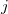] find theth excited state with the total spin of;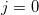means the SCF ground state. RECOMMENDATION:is ignored for restricted calculations; for unrestricted calculations,can only be 0 or 1. MECP_STATE2  Determines the second state for crossing. TYPE:  INTEGER/INTEGER ARRAY DEFAULT:  None OPTIONS:  [,] find theth excited state with the total spin of;means the SCF ground state. RECOMMENDATION:is ignored for restricted calculations; for unrestricted calculations,can only be 0 or 1. CIS_S2_THRESH  Determines whether a state is singlet or triplet in unrestricted calculations. TYPE:  INTEGER DEFAULT:  120 OPTIONS:  None RECOMMENDATION:  If set to 120, the states with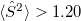are treated as triplet states, with other states are treated as singlets. MECP_PROJ_HESS  Determines whether to project out the coupling vector from the Hessian when using branching plane updating method. TYPE:  LOGICAL DEFAULT:  TRUE OPTIONS:  TRUE FALSE RECOMMENDATION:  Use Default. ## 9.4.2 Examples The MECP between the Sand Sstates of NO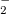is optimized using the direct method: Example 9.192 MECP optimization for NOusing SOS-CIS(D0) $MOLECULE
-1 1
N1
O2 N1 RNO
O3 N1 RNO O2 AONO

RNO=1.50
AONO=100
$END$rem
jobtype         = opt
method          = soscis(d0)
basis           = aug-cc-pVDZ
aux_basis       = rimp2-aug-cc-pVDZ
purecart        = 1111
cis_n_roots     = 4
cis_triplets    = false
cis_singlets    = true
mem_static      = 900
mem_total       = 1950
mecp_opt true
mecp_state1 [0,2]
mecp_state2 [0,3]
mecp_methods mecp_direct
$end  The MECP between the Sand Sstates of ethylidene is optimized using the branching-plane update method: Example 9.193 MECP optimization for ethylidene using SF-TDDFT $molecule
0 3
C  0.0446266041 -0.2419241370 0.3571573801
C  0.0089051507 0.6727548956 1.4605006396
H  0.9284257388 -0.1459163900 -0.2720952334
H -0.8310326564 -0.1926895078 -0.2885298629
H -0.0092388670 0.9611331703 2.4799363398
H  0.0683140308 -1.2533580302 0.7788470826
$end$rem
jobtype       opt
mecp_opt      true
mecp_methods  branching_plane
MECP_PROJ_HESS true !project out y vector from the hessian
GEOM_OPT_COORDS 0  !currently only works for Cartesian coordinate

method        bhhlyp
spin_flip     true
unrestricted  true
basis         6-31G(d,p)
cis_n_roots   4
mecp_state1   [0,1]
mecp_state2   [0,2]
CIS_S2_THRESH 120
$end  The MECP between Sand Sstates of twisted-pyramidalized ethylene is optimized using the penalty-constrained method: Example 9.194 MECP optimization for ethylene using SF-TDDFT $molecule
0 3
C -0.0158897609 0.0735325545 -0.0595597308
C  0.0124274563 -0.0024687284 1.3156941918
H  0.8578762360 0.1470146857 -0.7105293671
H -0.9364708648 -0.0116961121 -0.6267613144
H  0.7645577838 0.6633816890 1.7625731128
H  0.7407739370 -0.8697640880 1.3285831079
$end$rem
jobtype       opt
mecp_opt      true
mecp_methods  PENALTY_FUNCTION

method        bhhlyp
spin_flip     true
unrestricted  true
basis         6-31G(d,p)
cis_n_roots   4
mecp_state1   [0,1]
mecp_state2   [0,2]
CIS_S2_THRESH 120
$end  The MECP between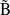Aand ÃBstates of the N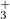ion using the direct method: Example 9.195 MECP optimization for Nusing EOM-EE-CCSD $MOLECULE
1 1
N1
N2 N1 rNN
N3 N2 rNN N1 aNNN

rNN=1.54
aNNN=50.0
$END$REM
JOBTYPE               OPT
MECP_OPT              TRUE
MECP_METHODS          mecp_direct

METHOD                EOM-CCSD
BASIS                 6-31G

ee_singlets           [0,2,0,2]

XOPT_STATE_1          [0,2,2]
XOPT_STATE_2          [0,4,1]
ccman2                false

$END  MECP optimization using analytic derivative coupling: Example 9.196 MECP betweenandof ethylidene optimized using BH&HLYP spin-flip TDDFT with analytic derivative coupling $molecule
0 3
C  0.0446266041 -0.2419241370 0.3571573801
C  0.0089051507 0.6727548956 1.4605006396
H  0.9284257388 -0.1459163900 -0.2720952334
H -0.8310326564 -0.1926895078 -0.2885298629
H -0.0092388670 0.9611331703 2.4799363398
H  0.0683140308 -1.2533580302 0.7788470826
$end$rem
jobtype opt
mecp_opt      true
mecp_methods  branching_plane
MECP_PROJ_HESS true
GEOM_OPT_COORDS 0
mecp_state1  [0,1]
mecp_state2  [0,2]
unrestricted true
spin_flip true
cis_n_roots 4
cis_der_couple true
CIS_DER_NUMSTATE 2
set_iter 50
exchange bhhlyp
basis 6-31G(d,p)
symmetry_ignore true
\$end